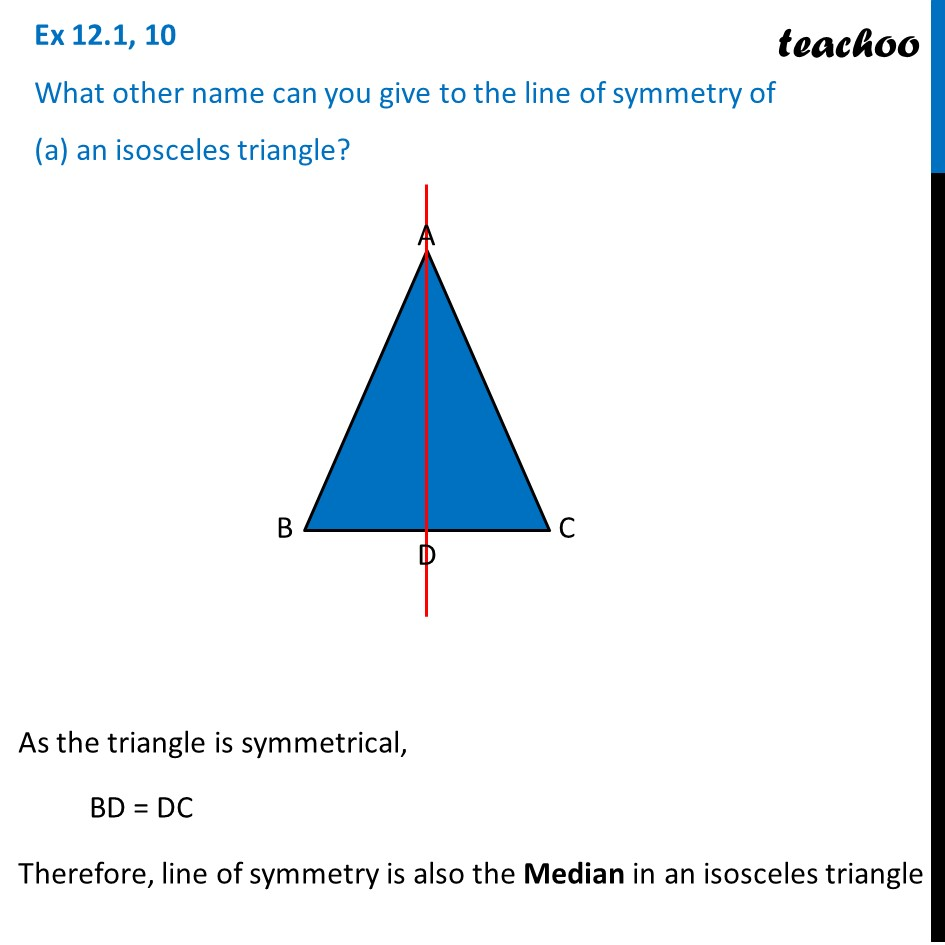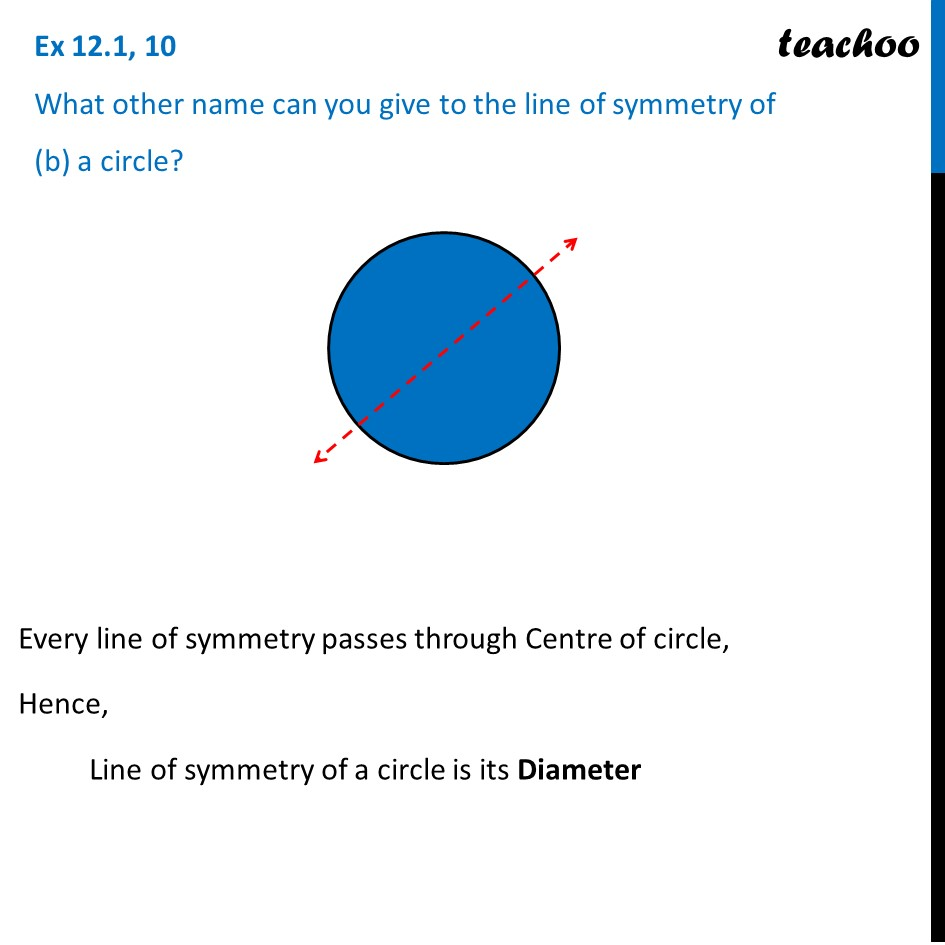Ex 12.1

Chapter 12 Class 7 Symmetry
Serial order wiseLearn in your speed, with individual attention - Teachoo Maths 1-on-1 Class

### Transcript

Ex 12.1, 10 What other name can you give to the line of symmetry of (a) an isosceles triangle? As the triangle is symmetrical, BD = DC Therefore, line of symmetry is also the Median in an isosceles triangle Ex 12.1, 10 What other name can you give to the line of symmetry of (b) a circle? Every line of symmetry passes through Centre of circle, Hence, Line of symmetry of a circle is its Diameter